# Geodesic curvature

at a point of a curve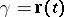on a surfaceThe rate of rotation of the tangent toaround the normal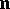to, i.e. the projection onof the vector of the angular rate of rotation of the tangent moving along. It is assumed thatandare regular and oriented, and that the velocity is taken relative to the arc lengthalong. The geodesic curvature can be defined as the curvature of the projection ofon the plane tangent toat the point under consideration. The geodesic curvature is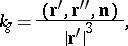where a prime denotes differentiation with respect to.

The geodesic curvature forms part of the function expressing the variation of the lengthasis varied on. If the ends are fixed: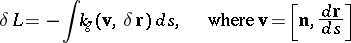and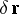is the vector of variation of the curve. Curves for which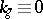are geodesic lines (cf. Geodesic line).

The integral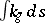is called the total geodesic curvature, or the rotation, of the curve. The connection between the rotation of a closed contour and the total curvature of the included region on the surface is given by the Gauss–Bonnet theorem.

The geodesic curvature forms a part of the interior geometry of the surface, and can be expressed in terms of the metric tensor and the derivatives of the intrinsic surface coordinates with respect to its parameter. If the geometry of a Riemannian space is studied without considering the latter to be immersed in Euclidean space, then the geodesic curvature is the only curvature which can be defined for a curve and the word "geodesic" is omitted. In considering curves on a submanifold of a Riemannian space, the curvature of a curve may be defined in the external space and in the submanifold — just like the spatial curvature and the geodesic curvature of a curve on a surface.

The concept of the geodesic curvature may be introduced for a curveon a general convex surface. If the curvehas a length and each one of its arcs has a certain rotation, the right (left) geodesic curvature ofat a pointis the limit of the ratio of the right (left) rotation of the arc to its length, under the condition that the arc is contracted towards the point.

Two concepts of geodesic curvature are defined in a Finsler space. These differ in the manner in which the length of the vector replacingis determined. On geodesics these geodesic curvatures are zero.